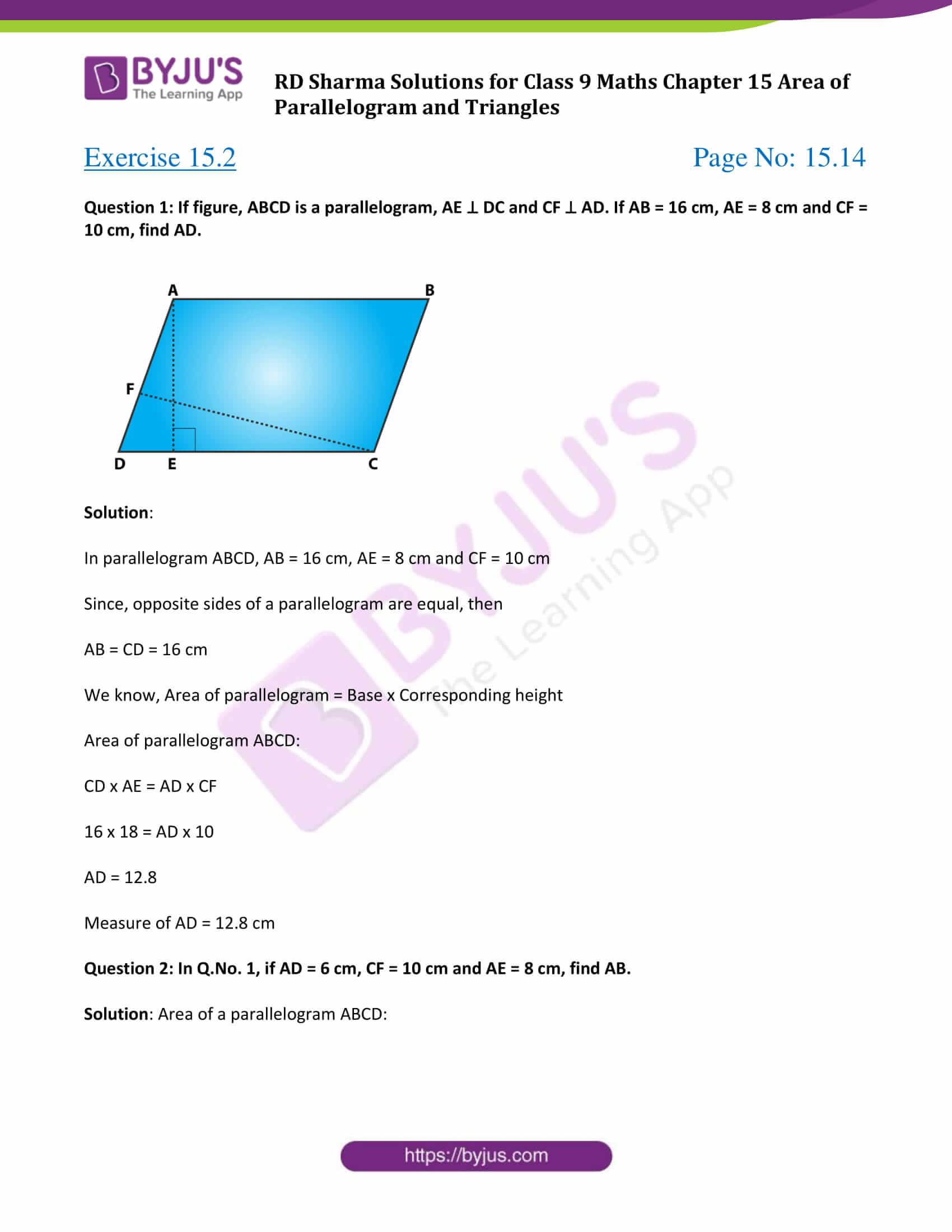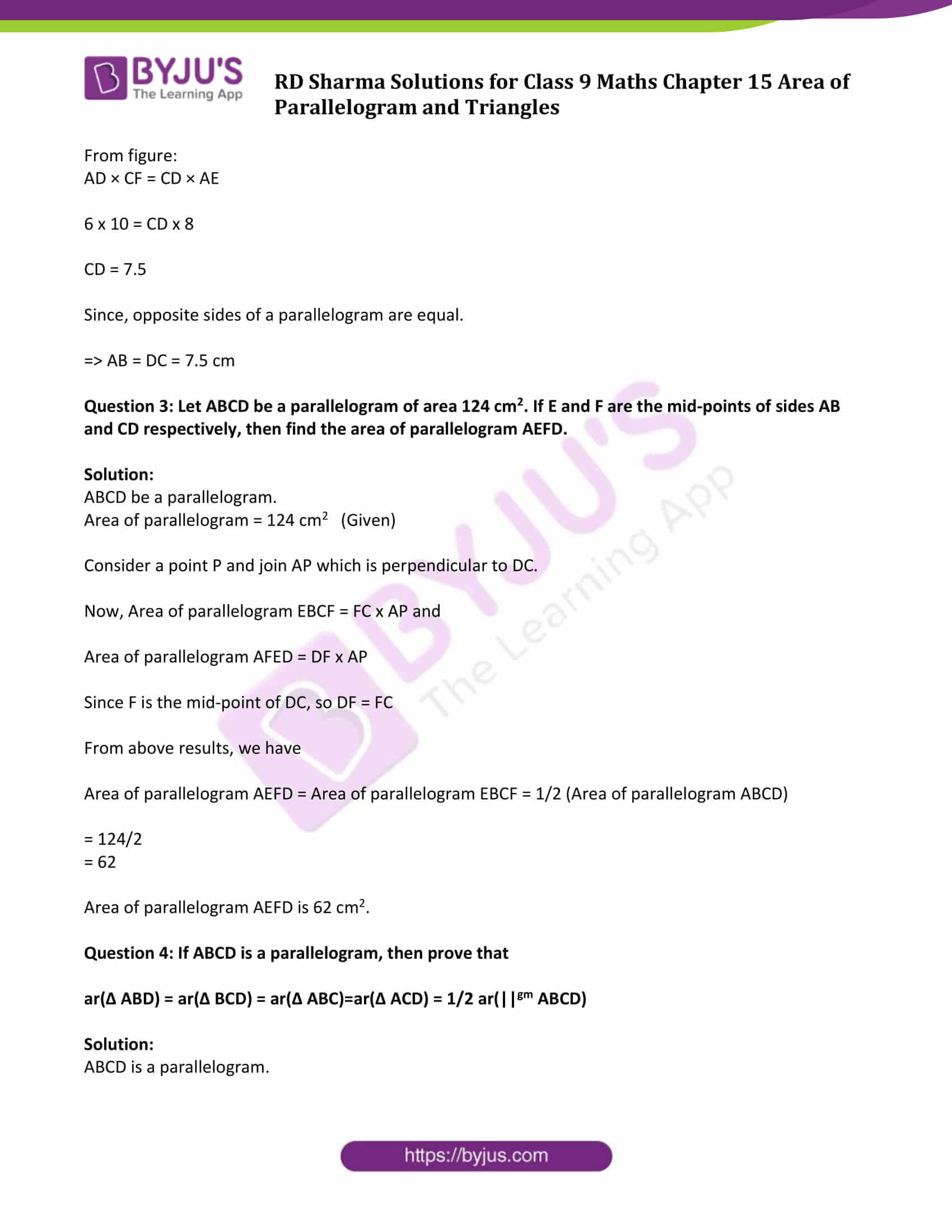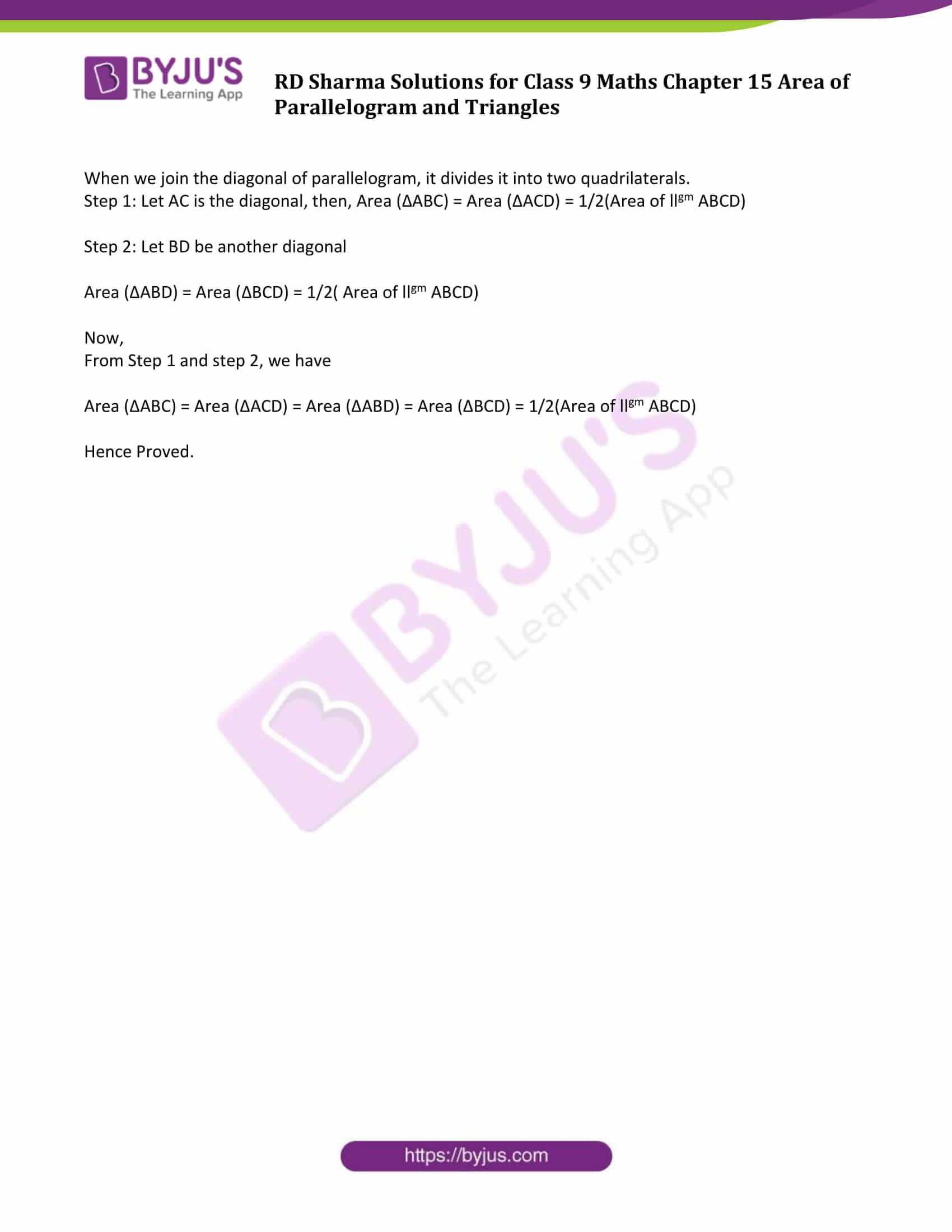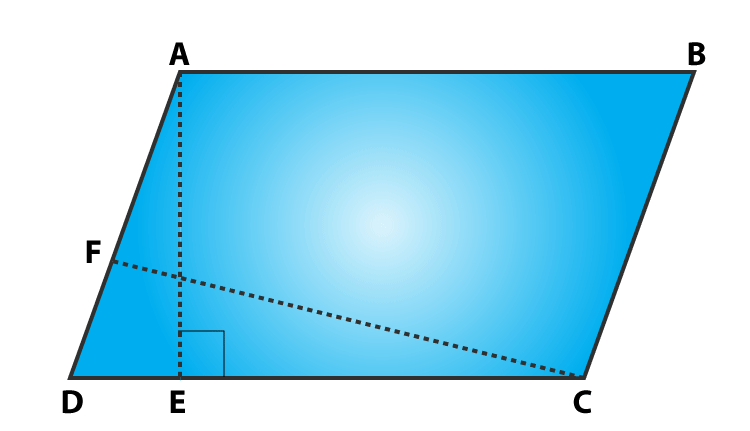# RD Sharma Solutions for Class 9 Maths Chapter 15 Area of Parallelograms and Triangles Exercise 15.2

Get free RD Sharma Solutions for Class 9 Maths Chapter 15 exercise 15.2 Area of Parallelograms and Triangles here. This exercise deals with problems based on polygon region and area axioms. Learn how to calculate Areas of Parallelograms and Triangles, through solved RD Sharma Solutions that are provided in this section.

## Download PDF of RD Sharma Solutions for Class 9 Maths Chapter 15 Area of Parallelograms and Triangles Exercise 15.2### Access Answers to Maths RD Sharma Solutions for Class 9 Chapter 15 Area of Parallelograms and Triangles Exercise 15.2 Page number 15.14

Question 1: If figure, ABCD is a parallelogram, AE ⊥ DC and CF ⊥ AD. If AB = 16 cm, AE = 8 cm and CF = 10 cm, find AD.Solution:

In parallelogram ABCD, AB = 16 cm, AE = 8 cm and CF = 10 cm

Since, opposite sides of a parallelogram are equal, then

AB = CD = 16 cm

We know, Area of parallelogram = Base x Corresponding height

Area of parallelogram ABCD:

CD x AE = AD x CF

16 x 18 = AD x 10

Measure of AD = 12.8 cm

Question 2: In Q.No. 1, if AD = 6 cm, CF = 10 cm and AE = 8 cm, find AB.

Solution: Area of a parallelogram ABCD:

From figure:

AD × CF = CD × AE

6 x 10 = CD x 8

CD = 7.5

Since, opposite sides of a parallelogram are equal.

=> AB = DC = 7.5 cm

Question 3: Let ABCD be a parallelogram of area 124 cm2. If E and F are the mid-points of sides AB and CD respectively, then find the area of parallelogram AEFD.

Solution:

ABCD be a parallelogram.

Area of parallelogram = 124 cm2 (Given)

Consider a point P and join AP which is perpendicular to DC.

Now, Area of parallelogram EBCF = FC x AP and

Area of parallelogram AFED = DF x AP

Since F is the mid-point of DC, so DF = FC

From above results, we have

Area of parallelogram AEFD = Area of parallelogram EBCF = 1/2 (Area of parallelogram ABCD)

= 124/2

= 62

Area of parallelogram AEFD is 62 cm2.

Question 4: If ABCD is a parallelogram, then prove that

ar(Δ ABD) = ar(Δ BCD) = ar(Δ ABC)=ar(Δ ACD) = 1/2 ar(||gm ABCD)

Solution:

ABCD is a parallelogram.

When we join the diagonal of parallelogram, it divides it into two quadrilaterals.

Step 1: Let AC is the diagonal, then, Area (ΔABC) = Area (ΔACD) = 1/2(Area of llgm ABCD)

Step 2: Let BD be another diagonal

Area (ΔABD) = Area (ΔBCD) = 1/2( Area of llgm ABCD)

Now,

From Step 1 and step 2, we have

Area (ΔABC) = Area (ΔACD) = Area (ΔABD) = Area (ΔBCD) = 1/2(Area of llgm ABCD)

Hence Proved.

## RD Sharma Solutions for Class 9 Maths Chapter 15 Area of Parallelograms and Triangles Exercise 15.2

RD Sharma Solutions Class 9 Maths Chapter 15 Area of Parallelograms and Triangles Exercise 15.2 is based on the following topics and subtopics:

Polygon Regions

Triangular region

Rectangular region

Polygon region

Area Axioms

-Area Axiom

-Congruent area axiom

-Area monotone axiom